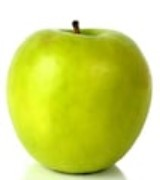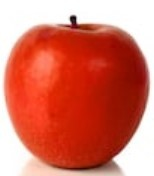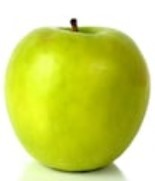# Measure similarity between images using Python-OpenCV

Prerequisites: Python OpenCV

Suppose we have two data images and a test image. Let’s find out which data image is more similar to the test image using python and OpenCV library in Python.

Let’s first load the image and find out the histogram of images.

Importing library

`import cv2`

Importing image data

`image = cv2.imread('test.jpg')`

Converting to gray image

`gray_image = cv2.cvtColor(image, cv2.COLOR_BGR2GRAY)`

Finding Histogram

```histogram = cv2.calcHist([gray_image], ,
None, , [0, 256])```

Example:

Images used:

data1.jpgdata2.jpgtest.jpg`import` `cv2 ` `  `  `     `  `# test image ` `image ``=` `cv2.imread(``'cat.jpg'``) ` `gray_image ``=` `cv2.cvtColor(image, cv2.COLOR_BGR2GRAY) ` `histogram ``=` `cv2.calcHist([gray_image], [``0``],  ` `                         ``None``, [``256``], [``0``, ``256``]) ` `  `  `# data1 image ` `image ``=` `cv2.imread(``'cat.jpeg'``) ` `gray_image1 ``=` `cv2.cvtColor(image, cv2.COLOR_BGR2GRAY) ` `histogram1 ``=` `cv2.calcHist([gray_image1], [``0``],  ` `                          ``None``, [``256``], [``0``, ``256``]) ` `  `  `# data2 image ` `image ``=` `cv2.imread(``'food.jpeg'``) ` `gray_image2 ``=` `cv2.cvtColor(image, cv2.COLOR_BGR2GRAY) ` `histogram2 ``=` `cv2.calcHist([gray_image2], [``0``],  ` `                          ``None``, [``256``], [``0``, ``256``]) ` `  `  `  `  `c1, c2 ``=` `0``, ``0` `  `  `# Euclidean Distace between data1 and test ` `i ``=` `0` `while` `i<``len``(histogram) ``and` `i<``len``(histogram1): ` `    ``c1``+``=``(histogram[i]``-``histogram1[i])``*``*``2` `    ``i``+``=` `1` `c1 ``=` `c1``*``*``(``1` `/` `2``) ` `  `  ` `  `# Euclidean Distace between data2 and test ` `i ``=` `0` `while` `i<``len``(histogram) ``and` `i<``len``(histogram2): ` `    ``c2``+``=``(histogram[i]``-``histogram2[i])``*``*``2` `    ``i``+``=` `1` `c2 ``=` `c2``*``*``(``1` `/` `2``) ` `  `  `if``(c1

Output :

`data1.jpg is more similar to test.jpg as compare to data2.jpg`

My Personal Notes arrow_drop_upCheck out this Author's contributed articles.

If you like GeeksforGeeks and would like to contribute, you can also write an article using contribute.geeksforgeeks.org or mail your article to contribute@geeksforgeeks.org. See your article appearing on the GeeksforGeeks main page and help other Geeks.

Please Improve this article if you find anything incorrect by clicking on the "Improve Article" button below.

Article Tags :

Be the First to upvote.

Please write to us at contribute@geeksforgeeks.org to report any issue with the above content.